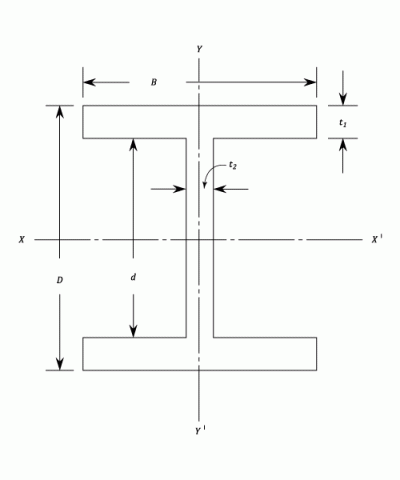•http://facebook.com/
•https://www.google.com/accounts/o8/id
•https://me.yahoo.com

# Moments of Inertia

Determination of Moments of Inertia by both analytical and graphical methods.

## Moments Of Inertia

The moment of Inertia ,also called the second moment of area, is defined as either
or
depending upon which axis the moment is taken.

It can be shown that the sum of and equals (the Polar Moment of Inertia). This relationship is called the Perpendicular Axis Theorem which states that the sum of the moments of area about any two axes in the plane is equal to the moment of inertia about the axis perpendicular to the plane, the three axes being concurrent.

It follows that the sum of the moments of inertia about any two perpendicular axes through a given point in the plane is constant.

## Circular Section

Inertia is the resistance of any physical object to a change in its state of motion or rest, or the tendency of an object to resist any change in its motion. It is proportional to an object's mass.

To calculate the polar moment of inertia about the centre of the section :

But and by the parallel axis theory (For proof of theorem see next section), and since and are equal being moments of inertia about a diameter.

For a hollow section with external diameters and internal diameters of and .

and

The ratio max is called the section modulus , so that the maximum stress equals .

The bending moment which can be carried by a given section for a limiting stress is called the Moment of resistance.

A bending moment exists in a structural element when a moment is applied to the element so that the element bends.

## The Parallel Axis Theorem

(by definition)

since
Stated in words:

The moment of inertia about any axis is equal to the moment of inertia about a parallel axis through the centroid plus the area times the square of the distance between the axes.

It should be noted that the moment of inertia through the centroid is the minimum value for any axis in that particular direction.

If it is required to transfer from one axis to a parallel axis , neither being through the centroid, then the operation must be done in two stages.

i.e. And

From which

## Calculations Of Moments Of Inertia

a) Rectangular section.

From the diagram,

For a hollow section of outside dimensions and inside dimensions

b) section

In the case of standard sections the moments of inertia are computed graphically from the actual shape of the cross section as rolled. However, a reasonable approximation can be made by estimating the mean flange thickness and working from a series of rectangles.Using the dimensions shown, the moment of inertia about may be obtained by subtracting that for rectangles wide and deep from the overall figure for ties .

i.e.

Alternatively, for greater accuracy of calculation, the web and flanges may be treated separately using the parallel axis theorem for the flanges.

Hence,

Where is the distance between the centroid axis of the flange itself and the principle axis of the whole cross section . The term is very small and can usually be neglected

The width being the dimension parallel to and the depth parallel to .

## The Graphical Determination Of Moment Of Inertia.

Assume that it is required to find the Moment of Inertia of an irregular shape about its centroid, .

Let be a convenient axis Outside the section. Note is parallel to .

Divide the Shape up into strips of area which are parallel to and at a distance from it. As each strip is of the same width , the areas will be proportional to their width .

It is now possible to complete the following table: